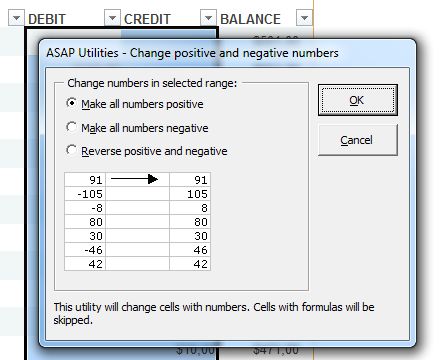Tip: Quickly make numbers positive or negative

Save 5 minutes a day by using ASAP Utilities to quickly change the sign of numbers for you.

"Easily change the sign of numbers"

Sometimes when you're working with amounts or if you have copied data from several sources, you need to change the negative numbers to positive or the other way around. Instead of using helper columns with formulas to do this, just let ASAP Utilities do it for you.

Just select the numbers and then start the following tool:
ASAP Utilities » Numbers & Dates » Change negative numbers to positive and vice versa...You can choose to:

1. Make all numbers positive
2. Make all numbers negative
3. Reverse positive and negative.
This will make all positive numbers negative, and all negative numbers positive.

Do you recognize any of these situations?

• How do I turn all numbers in one column to negative in Excel, without manually placing a negative sign before each number?
• I tried using the replace function- but could not figure out how to change a positive amount to a negative amount easily...
• In Excel, how can I automatically change all negative numbers in 4 columns to positive numbers? I do not wish to manually change all these numbers since it is a very long list!
• How do I convert numbers to the opposite sign? I need to change all positive numbers in the spreadsheet to negative numbers, and vice versa in Excel.
• I would like to change the sign (from (-) to (+) and from (+) to (-)) in my data. I would like to change the sign of columns C, D and I. Any idea how this can be done?

Just use the following tool:
ASAP Utilities » Numbers & Dates » Change negative numbers to positive and vice versa...

This is a quicker and easier solution than using temporary columns with formulas to multiply by -1 or using Excel's =ABS() formula or using Excel's Paste Special > Multiply trick.

How much time will it save?

It's guaranteed that you'll save yourself time and effort by using this tool. However, the actual time saved depends on how much you use Excel, the amount of data you're working with and how often you use this particular tool.
You can easily see how much time ASAP Utilities has saved you so far.